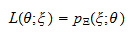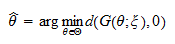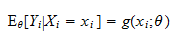StatLect

Estimation methods

In the lecture entitled Point estimation we have defined the concept of an estimator and we have discussed criteria to evaluate estimators, but we have not discussed methods to derive estimators. This lecture discusses general techniques that can be used to derive parameter estimators in a parametric estimation problem.

Before starting, let us recall the main elements of a parametric estimation problem:

• a sampleis used to make statements about the probability distribution that generated the sample;

• the sampleis regarded as the realization of a random vector, whose unknown joint distribution function, denoted by, is assumed to belong to a set of distribution functions, called statistical model;

• the modelis put into correspondence with a setof real vectors;is called the parameter space and its elementsare called parameters;

• the parameter associated with the unknown distribution functionthat actually generated the sample is denoted byand it is called the true parameter (if several different parameters are put into correspondence with,can be any one of them);

• a predefined rule (a function) that associates a parameter estimateto eachin the support ofis called an estimator (the symbolis often used to denote both the estimate and the estimator and the meaning is usually clear from the context).Extremum estimators

Several widely employed estimators fall within the class of extremum estimators. An estimatoris an extremum estimator if it can be represented as the solution of a maximization problem:whereis a function of both the parameterand the sample.

General conditions can be derived for the consistency and asymptotic normality of extremum estimators. We do not discuss them here (see, e.g., Hayashi, F. (2000) Econometrics, Princeton University Press), but we rather give some examples of extremum estimators and we refer the reader to lectures that describe these examples in a more detailed manner.

Maximum likelihood

In maximum likelihood estimation, we maximize the likelihood of the sample:where:

1. ifis discrete, the likelihoodis the joint probability mass function ofassociated to the distribution that corresponds to the parameter;

2. ifis absolutely continuous, the likelihoodis the joint probability density function ofassociated to the distribution that corresponds to the parameter.is called the maximum likelihood estimator of.

Maximum likelihood estimation is discussed in more detail in the lecture entitled Maximum Likelihood.

Generalized method of moments

In generalized method of moments (GMM) estimation, the distributions associated to the parametersare such that they satisfy the moment condition:whereis a (vector) function andindicates that the expected value is computed using the distribution associated to. The GMM estimatoris obtained aswhereis a measure of the distance offrom its expected value ofand the estimator is an extremum estimator becauseLeast squares

In least squares estimation the samplecomprisesrealizations, ...,of a random variable, called the dependent variable, andobservations, ...,of a random vector, whose components are called independent variables. It is postulated that there exists a functionsuch thatThe least squares estimatoris obtained asThe estimator is an extremum estimator becauseThe book

Most of the learning materials found on this website are now available in a traditional textbook format.

Glossary entries
Share